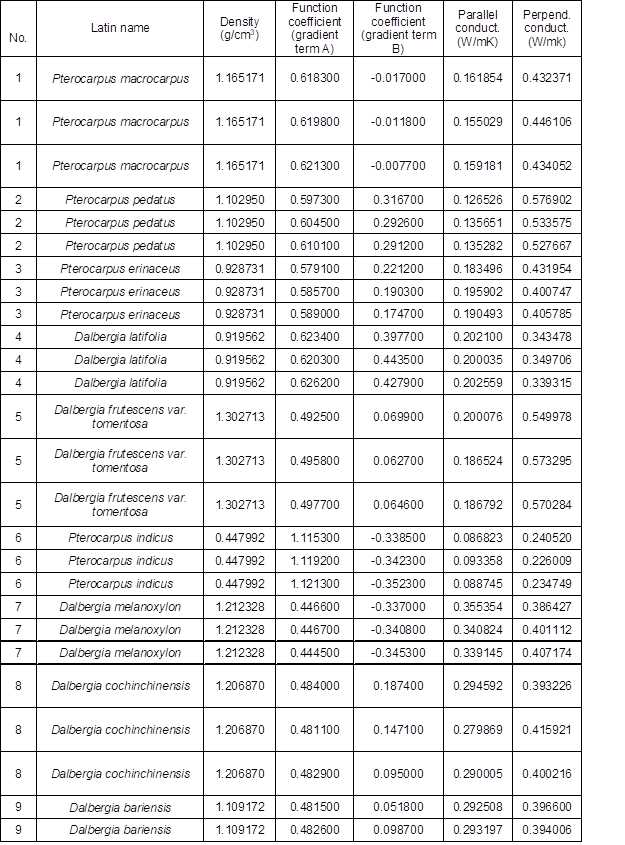Zhou, S., Yang, X., Zhang, Y., Liu, X., and Zhou, Y. (2021). "Prediction of the thermal conductivities of wood based on an intelligent algorithm," BioResources 16(1), 1161-1185.

#### Abstract

For thermal comfort and energy-saving performance, a floor-heating method is superior to conventional heating modes, e.g., radiator, fan coil, etc. The floor-heating method has been developed to be a primary indoor heating form. Wood is the most common floor surface material. Due to the anisotropy of wood, it is difficult to obtain a general theoretical formula for its thermal physical properties. In this paper, intelligent algorithms were adopted to predict thermal conductivities of wood. First, the study elaborated frequently used testing methods of thermal conductivity. Next, 130 types of common wood species were measured to form a database of thermal properties. With this database, intelligent algorithms were used to make predictions. For the thermal conductivity predictions that were conducted with support vector machine, the degree of fit between the predicted results and the measured results was not less than 0.87 (k-fold validation). This study validated the feasibility of the usage of the intelligent algorithm for the research and prediction of the thermal conductivities of wood.

Prediction of the Thermal Conductivities of Wood Based on an Intelligent Algorithm

Shiyu Zhou,a,* Xiaoxia Yang,Yandong Zhang,b Xiaoping Liu,b and Yucheng Zhou b

For thermal comfort and energy-saving performance, a floor-heating method is superior to conventional heating modes, e.g., radiator, fan coil, etc. The floor-heating method has been developed to be a primary indoor heating form. Wood is the most common floor surface material. Due to the anisotropy of wood, it is difficult to obtain a general theoretical formula for its thermal physical properties. In this paper, intelligent algorithms were adopted to predict thermal conductivities of wood. First, the study elaborated frequently used testing methods of thermal conductivity. Next, 130 types of common wood species were measured to form a database of thermal properties. With this database, intelligent algorithms were used to make predictions. For the thermal conductivity predictions that were conducted with support vector machine, the degree of fit between the predicted results and the measured results was not less than 0.87 (k-fold validation). This study validated the feasibility of the usage of the intelligent algorithm for the research and prediction of the thermal conductivities of wood.

Keywords: Wood; Floor heating; Support Vector machine; Thermal conductivity

Contact information: a: School of Thermal Engineering, Shandong Jianzhu University, Jinan, Shandong 250101 China; b: School of Information and Electrical Engineering, Shandong Jianzhu University, Jinan, Shandong 250101 China; *Corresponding author: zhou-1sy@163.com

INTRODUCTION

Since the supplying water temperature of a floor heating system is lower than that of a conventional heating system, such as a radiator, its energy consumption can be provided by renewable energies, e.g., solar energy, air source, and shallow geothermal energy. This characteristic allows floor heating systems to save greater amounts of energy, leading to its popularity. The study by Márquez et al. (2017) compared and analyzed the heating effects of different end forms of heating systems, i.e., fan-coil, floor heating, and a combination of the two. The results showed that a floor heating system is superior in terms of both energy consumption and indoor comfort. Zhou and He (2015) conducted experiments on wooden floor heating systems with different heat storage materials and different heating pipes. Research by Shin et al. (2015) showed that the floor surface temperature distribution of oak wood was more even than other covering panels, such as linoleum, which means that wooden surfaces can provide a more comfortable indoor heating environment. The heating performance of wooden floors is closely related to the thermal properties of wood. The recent studies about this theme have concentrated on the thermal conductivity of wood. The publications have been primarily divided into theoretical studies and experimental studies:

1) Theoretical studies include model formulas and empirical formulas, e.g., calculation models of the basic structural units of wood. Research involving the calculation model of the effective thermal conductivity of wood has gradually developed from the earlier empirical formula to the calculation model of the basic structural units of wood. For example, in the study conducted by Thunman and Leckner (2002), measurable parameters were employed, e.g., density, moisture content, and shrinkage, to calculate the effective thermal conductivity of wood. They also developed a calculation model of the effective thermal conductivity at different combustion stages. A study by Guo et al. (2013) was based on the linear heat source model, which numerically calculated the effective thermal conductivity and specific heat capacity of bulk wood particles by the least square method. According to the experimental and numerical results, the empirical relationships between the effective thermal conductivity of wood particles and the water content, as well as the porosity were obtained. The results showed that the thermal conductivity had nothing to do with the size of the wood particles. Meanwhile, the empirical relationship between the specific heat capacity and the water content was obtained.

2) Experimental studies have been primarily conducted at the macroscale and microstructural levels. At the macroscale level, the studies primarily have concentrated on the effective thermal conductivity (neglecting the wood structure and composition). Wood is a typical porous material, and the heat transfer process in wood is the result of the heat transfer in the solid, liquid, and air phases that are present in the wood. Therefore, the thermal conductivity of porous materials such as wood is usually defined as an effective thermal conductivity or an apparent thermal conductivity. At the microscale level, the relevant studies have extended from the effective thermal conductivity to the real thermal conductivity. These studies have concentrated on the thermal properties of wood elements, such as the thermal conductivity of the cell walls. The study by Vay et al. (2013), focused on the heat conductivity properties of wood from a cellular view via scanning thermal microscopy (SThM). It was found that heat conductibility along the axis direction of the wood cell was higher than that of the cross-section direction. Fan et al. (2006) established a fractal dimension model of the effective thermal conductivity of horizontal grain wood, which was validated with experiments conducted via an improved transient plane source measurement method. Afterwards, Lagüela et al. (2015) measured the axial and radial heat conductivity of different wood specimens via the hot-disk transient technique. Their study demonstrated that the heat transfer rate was greatly affected by the direction of the wood grain. Since the measurements were conducted while the wood was in the drying state, moisture percentage effects were not considered.

In this study, 130 wood specimens, belonging to different families, were tested with a thermal constant analyzer in order to measure their thermal conductivities. Then, an intelligent model was established using support vector machines to predict the thermal conductivities of the tested wood specimens.

EXPERIMENTAL

Measuring Equipment and Theory

Experimental apparatus

The experiment was conducted with a thermal constant analyzer (TPS2200) produced by Hot Disk located in Gothenburg, Sweden, as shown in Fig. 1a. The analyzer can measure the volume specific heat, thermal conductivity, and thermal diffusion coefficient of different materials, e.g., metal, wood, plastic, etc. Since there is a mathematical relationship between the thermal conductivity and thermal diffusion coefficient, the two parameters can be measured with the same probe, as shown in Fig. 1b. The device is also equipped with a vessel for testing heat capacity, which is not figured in the following text.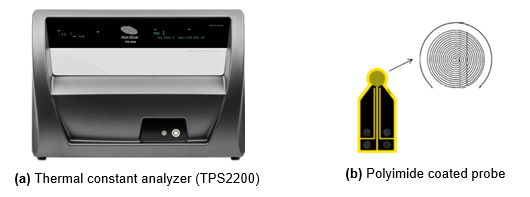Fig. 1. Experimental apparatus

Transient plane source method

The thermal conductivity and thermal diffusion coefficient were determined based on the transient plane source (TPS) method. Figure 2 is the test sketch map, and as shown, the probe was clamped above and below the tested specimen.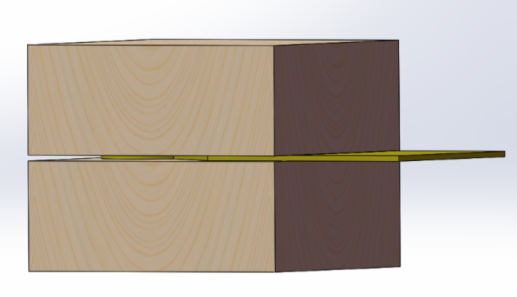Fig. 2. Sketch map of the thermal property measurement testing procedure

The specimens were processed into two parts with the same square shape prior to testing. As depicted in He (2005), the temperature distribution of the probe along with time and space can be expressed according to Eq. 1,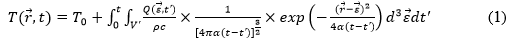where r is the radius, t is the time, is a function of space and time, Q is the power dissipation per unit volume [J/(s·m3)], T0 is the initial temperature, α is the thermal diffusion coefficient, ρ is the density, c is the thermal capacity, and V’ is the heat source volume. Equation 1 can be simplified according to Eq. 2,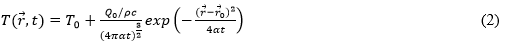where Q0 is the power of the heat source at the initial time [J/(s·m3)]. The mathematical relationship between the thermal conductivity and thermal diffusion coefficient is shown in Eq. 3,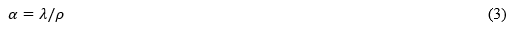As shown in Fig. 1b, the probe is a double helical structure and provides constant heating power. Therefore, the probe can be simplified into a series of isometric concentric circles for analysis purposes, in order to simplify the calculation. For a probe with a cylinder number of m and a maximum radius of a, the length of the metal wire is calculated according to Eq. 4,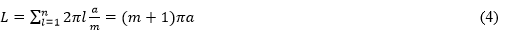On the basis of the above simplified analysis (Eq. 4), one of the single rings was taken for analysis. In the cylindrical coordinate, the coordinates of any point on the sample surface can be expressed as p=(r,θ,z) and the coordinates of any point on the metal ring can be written as q=(r’, θ’, z’). There is an equality relationship between the two coordinates, as demonstrated in Eq. 5,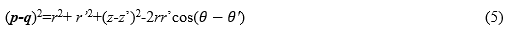At the plane of =0 on the cylindrical coordinate grid, temperature variation of the sample surface can be written as Eq. 6,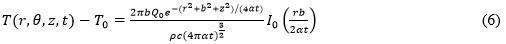where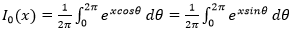is the first type of correctional Bessel function with zero order and is the radius of a single ring.

The output power of a single ring is supposed to be constant; therefore, when u(t’) is the unit step function, the mathematical equation of the output power is shown in Eq. 7,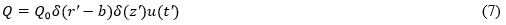Integration should be made towards to the output power of a single ring and the probe power can be formulated according to Eq. 8,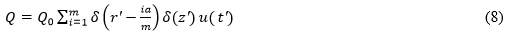Since the probe is defined at the surface of z’=0, the temperature rise equation of the probe surface can be written as Eq. 9,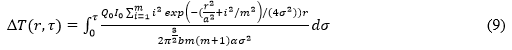where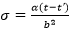represents the integration variable and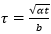is the dimensionless time constant. By searching for the mean values of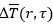, the temperature rise function can be formulated as Eq. 10,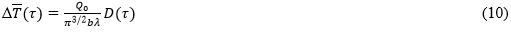where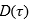is given by Eq. 11,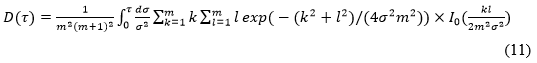It can be seen from Eq. 10 that there is a proportional relationship between the average surface temperature rise function of the probe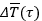and function of. When using the thermal constant analyzer, the thermal conductivity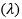can be computed with an equation betweenandwhereis the function of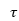. Therefore, when variables such as the number of wire loops is fixed, the value ofcan be computed with. In addition,is the function of , which can be calculated during the heating process. The computation theory can be clearly elaborated with the above formulations. However, there are also shortcomings that exist in the calculation process. It can be concluded from the above analysis thatcannot be directly obtained, because the value of is decided by the thermal diffusion coefficient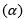, heating time (t), and probe radius (b), but the thermal diffusion coefficient is unknown, i.e., it needs to be measured. Therefore, it can only be computed via the iteration method, which is a time-consuming and complicated process. Therefore, in subsequent work it is intended to adopt the intelligent method for data processing and prediction works.

Data Collection and Preprocessing

Experimental data collection

This experiment measured 130 species of wood, which belonged to 39 different families (Table S1). The experimental specimen samples were processed from the original specimens, whose size was 210 mm x 105 mm x 20 mm (length by width by thickness). Figure 3(a) shows the processed specimen for measuring thermal conductivity and thermal diffusion coefficient, which was 50 mm x 50 mm x 20 mm (length by width by thickness). These specimens had been oven-dry to remove moisture before each test. So the influence of moisture content in the measured values was avoided.

Since the study was conducted under the context that applying wood floor to the floor heating system, the measurement directions were defined as shown in Fig. 3(b), rather than the real axial and radial directions of the wood. In this study, the two directions were defined according to the pavement of wood floor, the direction perpendicular to the floor was defined as ‘Perpendicular’, while the direction parallel to the floor is defined as ‘Parallel’. Thermal conductivities of the two directions were measured for the reason that ‘Perpendicular’ thermal conductivity affects the heat release to the room, which will determine the indoor temperature distribution, while ‘Parallel’ thermal conductivity affects the heat diffusion along the floor, which will determine the temperature uniformity of the floor.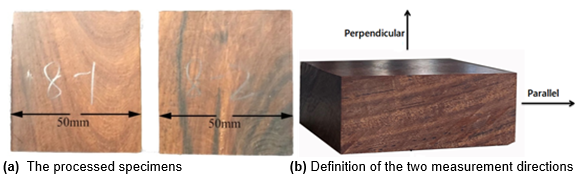Fig. 3. Specimens for measuring the thermal conductivity

Data preprocessing

It was stated in the above section that the thermal properties of wood can be computed from the transient temperature data. Therefore, it is necessary to extract features that can reflect the temperature-changing characteristics. For the study of the thermal conductivity, transient temperature rise diagram is necessary, as shown in Fig. 4.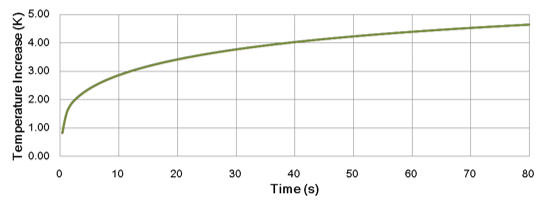Fig. 4. Transient temperature rise diagram of anisotropy thermal conductivity (Monterey pine)

According to the suggestions from the Hot Disk company, the number of gathered temperature data points of each test should be approximately 200. As described by the study objective in the first section, intelligent models for predicting the thermal properties of wood needed to be constructed in this study. If 200 data points are all set as model inputs, then it is almost impossible to obtain a valid and efficient model. In order to consider the regularity of the temperature change, feature extraction should be conducted towards to the temperature data. As shown in Fig. 4, the temperature curve was similar to the water temperature variations in the thermal response test (TRT) (Gehlin 2002). The data processing method of TRT, i.e., a logarithmic curve, was adopted for feature extraction, and the detailed linear fitting equation is shown as Eq. 12,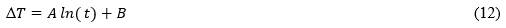where ∆T is the temperature increment, [K]; t is the time, [s]; and A and B are the coefficients that were extracted from the temperature process data. The measured data of 130 kinds of wood were processed with the above formula (Eq. 12). All of the degrees of fitness () values were larger than 0.98 and the partial fitting results are plotted in the following diagrams (as shown in Fig. 5).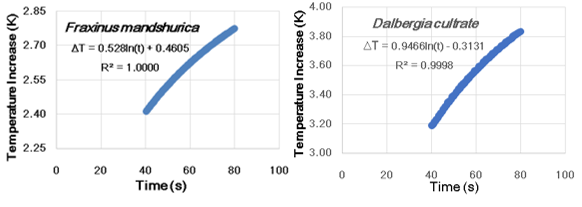Fig. 5. Transient temperature rise scatter diagrams of different specimens

Evaluation of the model accuracy should be calculated with several error indexes, e.g., the mean relative error (MRE), the max relative error (MAE), the mean square error (MSE), and the absolute fraction of variance (R2). The formulations of the above error indexes are expressed as Eqs. 15 through 18, respectively.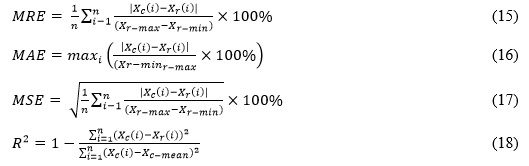where Xis the output of the model, Xc – mean is the average output of the model, Xis the experimental value, Xr– max is the maximum experimental value, and Xr – min is the minimum experimental value.

There are usually two validation ways, e.g., holdout validation and k-fold validation. For holdout validation: the data is split into training set and testing set, and then the model is trained and tested separately; while for k-fold validation, which is also called cycle validation. In the data preprocessing progress, the data is split into k subsets averagely. Then the training and testing processes are conducted for k times. In each time, one subset is defined as testing set for once, while the rest k-1 subsets are defined as training set. In turn, every subset can be treated as testing set for once. The prediction errors of each time are averaged to denote the final validation errors of the model.

Intelligent Algorithms

Many kinds of intelligent algorithms have been developed for the prediction or classification work in the last decades, such as Artificial Neural Network (ANN), Support Vector Machines (SVM), Adaptive Network-based Fuzzy Inference System (ANFIS) and Radial Basis Neural Network (RBN), etc. In these intelligent algorithms, SVM is commonly recognized to be suitable for the process of small data set. Because the number of experimental data is not so big, SVM was adopted for the prediction work in this study.

SVM is a machine learning algorithm basing on the statistical learning theory. Its principle is to construct the optimal separating hyperplane by mapping the input vector into a high-dimensional feature space according to the prior selected non-linear operator. SVM was born in the 90s of the last century; since then, it has been applied into more and more widely fields, such as predictions of outdoor/indoor temperature, wind speed, solar radiation, etc. (Paniagua-Tineo et al. 2011; Cai et al. 2015; Mohandes et al. 2004; Chen et al. 2011).

RESULTS AND DISCUSSION

Models for Predicting Thermal Conductivity

As mentioned in the above text, both ‘Perpendicular’ and ‘Parallel’ values of thermal conductivity need to be studied. Therefore, two separate models were trained in order to predict each of the two conductivity values. The two models have same input parameters, e.g., the sample density, together with the slope term (A) and intercept term (B) from Eq. 12. Although the two thermal conductivities were trained as outputs of two separate SVM models, their data was measured under the same testing conditions. The experiments were repeated 3 times and conducted under the following conditions: a heating power of 50 mW and an application period of 80 s. There were 390 sets of data generated from the experiment (as shown in Table S2), in which 360 of the sets were picked out at random to train the SVM model, while the remaining 30 sets were gathered for testing.

In the k-fold validations, the k value was selected as 13, meaning that the whole data was divided into 13 subsets averagely after the data sequence was disturbed, so as to keep the random selection of the training data. And then 13 times of training and validation process were conducted for each model. For the training parameters of SVM model, their values were given as ‘svmtrain(y_1_train,x_train,’-s 4 -t 2 -c 50 -g 180′)’.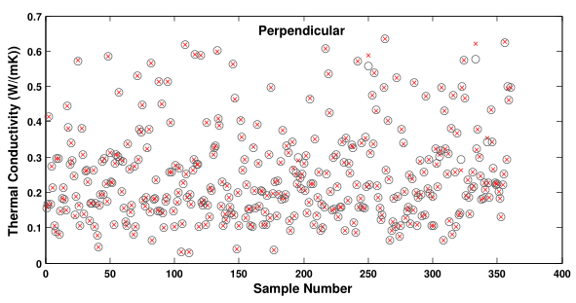Fig. 6. Training results of the Perpendicular thermal conductivity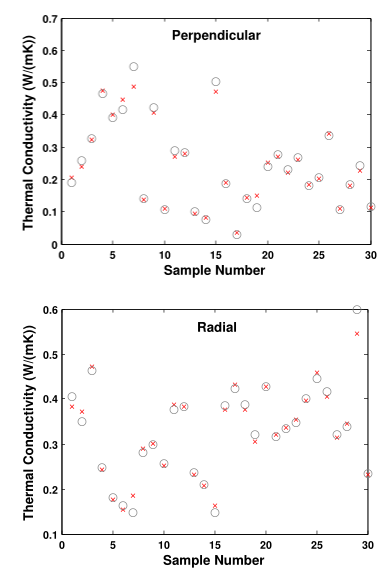Fig. 7. Validation results of the two thermal conductivities

The model prediction results are listed in Figs. 6 and 7. In these figures, the ‘x’ means the output of SVM model, and ‘○’ means an experimental value. The horizontal axis represents the sample number, while the vertical axis represents the thermal conductivity, whose unit is W/(m·K). Training results of the Perpendicular thermal conductivity are not shown here to save space. Good coincidence between prediction value and experimental value can be found in the figures. The detailed prediction errors are given in Table 1, which shows that the holdout validation results were superior to k-fold validation results. For both of the two validation methods, the degree of fitness of the training data sets was as high as 0.99, while the degree of fitness of the testing data sets was 0.87 or higher. The results demonstrated that the model was effective for an anisotropic thermal conductivity module.

Table 1. Prediction Errors of Thermal Conductivity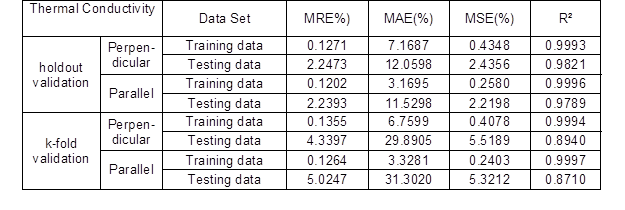CONCLUSIONS

1. The thermal properties of 130 species of wood that belonging to different families were tested with a Hot Disk thermal constant analyzer to generate the initial training database. The prediction works were then conducted towards to these woods based on intelligent algorithm.
2. SVM was adopted for the prediction of the thermal conductivity. In order to simplify the calculation process, feature extraction was adopted and separate models for predicting the Perpendicular and the Parallel thermal conductivity (relative to the floor) were established. The testing degrees of fitness of the two models both were at least 0.87, while holdout validation results were even better.
3. The success prediction of thermal conductivity with inputs of term A and B validated the fact that there may exists a mathematical relationship between thermal conductivity and , not only with , although the specific equations has not been found.

REFERENCES CITED

Cai, Q., Wang, W., and Wang, S. (2015). “Application of indoor temperature prediction based on SVM and BPNN,” Control & Decision Conference, 2883-2887. DOI:10.1109/CCDC.2015.7162418

Chen, J. L., Liu, H. B., Wu, W., and Xie, D. (2011). “Estimation of monthly solar radiation from measured temperatures using support vector machines – A case study,” Renewable Energy 36(1), 413-420. DOI: 10.1016/j.renene.2010.06.024

Fan, L.-W., Hu, Y.-C., Tian, T., and Yu, Z.-T. (2006). “The prediction of effective thermal conductivities perpendicular to the fibres of wood using a fractal model and an improved transient measurement technique,” International Journal of Heat and Mass Transfer 49(21-22), 4116-4123. DOI: 10.1016/j.ijheatmasstransfer.2006.03.027

Gehlin, S. (2002). Thermal Response Test Method Development and Evaluation, Ph.D. Dissertation, Lulea University of Technology, Luleå, Sweden

Guo, W., Lim, C. J., Bi, X., Sokhansanj, S., and Melin, S. (2013). “Determination of effective thermal conductivity and specific heat capacity of wood pellets,” Fuel 103, 347-355. DOI:10.1016/j.fuel.2012.08.037

He, Y. (2005). “Rapid thermal conductivity measurement with a hot disk sensor: Part 1. Theoretical considerations,” Thermochimica Acta 436(1-2), 122-129. DOI:10.1016/j.tca.2005.07.003

Lagüela, S., Bison, P., Peron, F., and Romagnoni, P. (2015). “Thermal conductivity measurements on wood materials with transient plane source technique,” Thermochimica Acta 600, 45-51. DOI:10.1016/j.tca.2014.11.021

Márquez, A. A., López, J. M. C., Hernández, F. F., Muñoz, F. D., and Andrés, A. C. (2017). “A comparison of heating terminal units: Fan-coil versus radiant floor, and the combination of both,” Energy and Buildings 138, 621-629. DOI:10.1016/j.enbuild.2016.12.092

Mohandes, M. A., Halawani, T. O., Rehman, S., and Hussain, A. A. (2004). “Support vector machines for wind speed prediction,” Renewable Energy 29(6), 939-947. DOI: 10.1016/j.renene.2003.11.009

Paniagua-Tineo, A., Salcedo-Sanz, S., Casanova-Mateo, C., Ortiz-Garcia, E. G., Cony, M. A., and Hernandez-Martin, E. (2011). “Prediction of daily maximum temperature using a support vector regression algorithm,” Renewable Energy 36, 3054-3060. DOI: 10.1016/j.renene.2011.03.030

Shin, M. S., Rhee, K. N., Ryu, S. R., Yeo, M. S., and Kim, K. W. (2015). “Design of radiant floor heating panel in view of floor surface temperatures,” Building and Environment 92, 559-577. DOI: 10.1016/j.buildenv.2015.05.006

Thunman, H., and Leckner, B. (2002). “Thermal conductivity of wood – Models for different stages of combustion,” Biomass and Bioenergy 23(1), 47-54. DOI:10.1016/S0961-9534(02)00031-4

Vay, O., Obersriebnig, M., Müller, U., Konnerth, J., and Gindl-Altmutter, W. (2013). “Studying thermal conductivity of wood at cell wall level by scanning thermal microscopy (SThM),” Holzforschung 67(2), 155-159. DOI:10.1515/hf-2012-0052

Zhou, G., and He, J. (2015). “Thermal performance of a radiant floor heating system with different heat storage materials and heating pipes,” Applied Energy 138(15), 648-660. DOI:10.1016/j.apenergy.2014.10.058

Article submitted: August 27, 2020; Peer review completed: October 18, 2020; Revised version received and accepted: November 26, 2020; Published: December 21, 2020.

DOI: 10.15376/biores.16.1.1161-1185

APPENDIX

Table S1. Detailed List of the Wood Specimens Measured in Experiment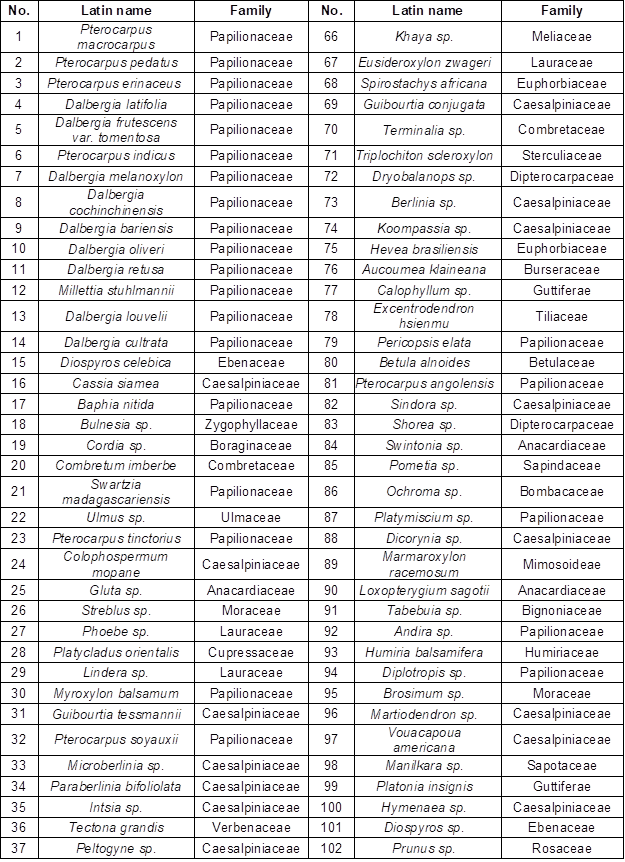Table S2. Listing of Density, Function Coefficient, Parallel Thermal Conductivity, and Perpendicular Thermal Conductivity